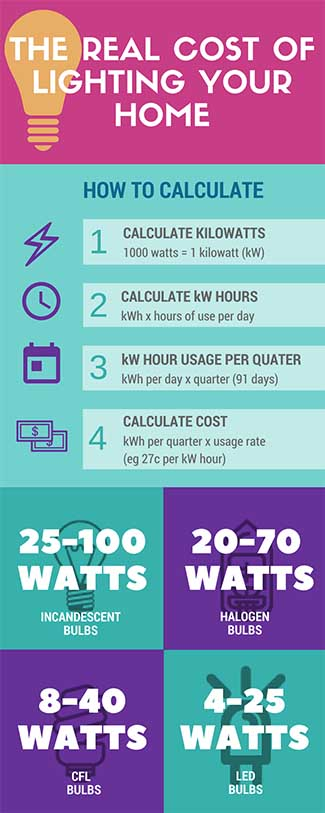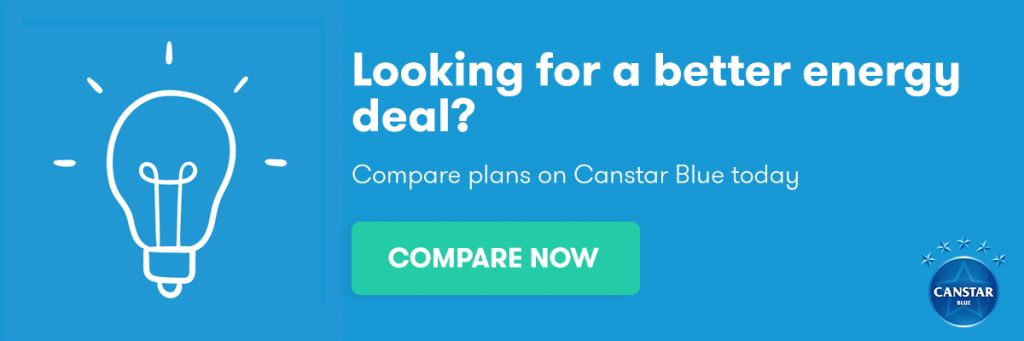# How much electricity do light bulbs use?

It’s easy to overlook how much your lights cost to run. After all, it can’t be that much, right?

Well, that depends on the types of bulbs you have installed. Here we take a look at four common types of residential light bulbs and see just how much they’re adding to your electricity bills.

## Types of light bulbs

There are a number of types of lights bulbs suitable for different applications, be it outdoor, downlight, lamps or ceiling. Focusing for the moment on interior ceiling lights, there are four types of light bulbs that you might find around a typical household – incandescent light bulbs, halogen light bulbs, compact fluorescent lights (CFLs) and light emitting diodes (LEDs).

• Incandescent bulbs: These light bulbs are simple and cheap, but very inefficient to run. The least efficient incandescent bulbs were progressively phased out since 2009, though you might still find some in an old property. An incandescent light can consume anywhere from 25 to 100 watts of electricity.
• Halogen: These bulbs are a great substitute for incandescent lights, with many bulbs coming in a similar shape and size.  Halogen lights are, however, notably more efficient and therefore have a lower wattage demand of anywhere from 20 to 70 watts.
• CFLs: These are those white spiralled bulbs, and are often considered to be the first truly efficient commercial lighting solution. Their wattage demand is usually only around eight to 40 watts.
• LEDs: These have blown up in popularity recently. While LED bulbs are more expensive to purchase, they’re generally the most efficient bulb, requiring little electricity to produce just as much light as other bulbs. An LED light will usually consume four to 25 watts.

## How much do lights cost to run?There are a few factors to consider here such as usage rates, bulb wattage and how often you use your bulbs. That means while we can’t accurately tell you how much your own lights are costing you, we can give you a basic run down on how to work it out for yourself.

### Calculating energy costs

Before we dive into light bulb running costs, here’s the basic math in four simple steps. To help illustrate, we’re going to start by using a large appliance, say a 300 watt television. A light bulb of course uses much less than that, but it’s easier to demonstrate the math with a larger number.

Step 1 – Calculate kilowatts:

Energy consumption of appliances and electronics is given in ‘watts’. The first step is to convert this to Kilowatts. To do this, you need to divide the wattage of your lightbulb by 1,000.

Watts/1,000 (example: 300 watts / 1000 = 0.3kW)

Step 2 – Calculate kilowatt hours:

Use the kW figure from step one to calculate kilowatt hours (kWh). Multiply kW by the number of hours of usage. Here we assume the TV is used for six hours per day.

kWh x hours of use per day (example: 0.3kW x six hours = 1.8kWh per day)

Step 3 – Calculate kilowatt hour usage per quarter:

A standard billing quarter is 91 days. All you need to do to solve your quarter usage is to multiply your daily usage by 91.

kWh per day x quarter period (example: 1.8 kWh/day x 91 days = 163.8kWh per quarter)

Step 4 – Calculate the cost:

For the last step, look at your electricity bill to see your usage rate. Let’s assume a rate of 27c per kilowatt hour.  For the quarterly cost of an appliance, multiply the usage rate by your total quarterly usage.

kWh per quarter x usage rate (example: 163.8kWh x 27c = \$44.23)

## So, how much will lighting cost?

The light output of bulbs is measured in lumens. Inefficient bulbs require more energy to produce the same number of lumens. Without going into too much detail here, to produce the same light output of say 720 lumens, the wattage required by different bulbs will be approximately as follows:

• Incandescent: 60 watts
• Halogen: 42 watts
• CFL: 15 watts
• LED: 12 watts

Now, using the equations we discussed above, we can work out how much each of these bulbs are costing to run. To compare, let’s take an extreme example that these bulbs are left running all day for the entire billing quarter (91 days). Again, we assume a flat electricity usage rate of 27c/kWh.

Light bulb Wattage Usage cost per quarter for ONE light bulb
Incandescent 60 \$35
Halogen 42 \$25
CFL 15 \$9
LED 12 \$7

Keep in mind that this doesn’t factor in the bulb purchase costs or how often you will need to replace them. Generally speaking, incandescent bulbs are the cheapest and have the shortest life, followed by halogen bulbs. On the other hand, while CFLs and LEDs tend to have greater efficiency and life span, they are a little pricier, with LED lights in particular being the most expensive type of bulb.

### The final word on lighting costs

While energy saving light bulbs are often more expensive upfront, most energy saving websites advocate that LED lights will usually save you money in the long term. According to energyrating.gov.au, the typical Aussie home has about 37 light bulbs, which can really add up in yearly electricity costs.

Even if you already consider yourself a rather savvy saver when it comes to electricity, it can’t hurt to do a quick energy audit of your home and ensure you’re using efficient light bulbs wherever possible.